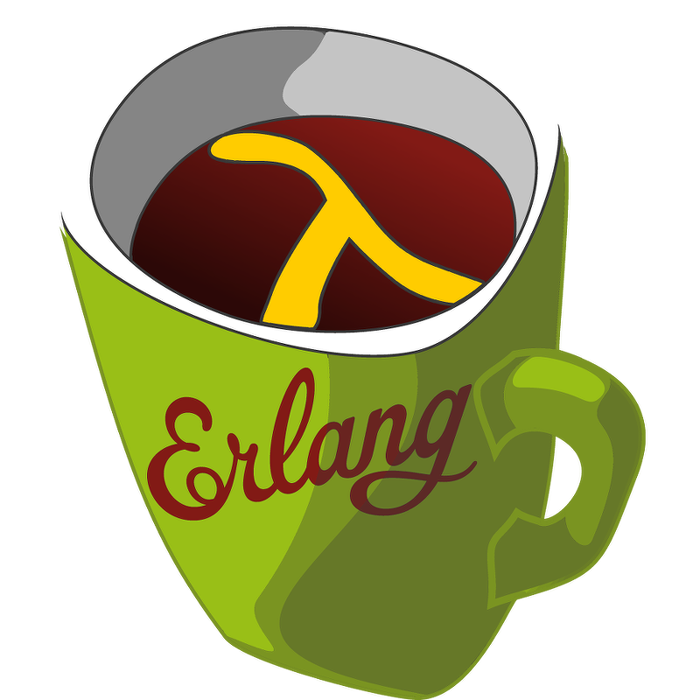This week's LFE Friday was translated with permission from the Erlang Thursday series by Steven Proctor. This week's translator: Robert Virding.

Today's LFE Friday starts to dig into the `digraph` module, as promised last week, and takes a look at digraph:add_vertex/1.

First we create a new directed graph, so we have something we can add vertices to.

``````> (set graph (digraph:new))
#(digraph 20495 24592 28689 true)``````

We then add some vertices to the graph by using `digraph:add_vertex/1`.

``````> (digraph:add_vertex graph)
(\$v . 0)
(\$v . 1)
(\$v . 2)``````

As we don't specify any information about the vertex we want to add, LFE will create a new vertex for us of the format `(\$v . i)`, with an empty list as the label where `i` is a non-negative integer.

We can also use `digraph:add_vertex/2` to add a vertex if we wish to provide the vertex identifier, or provide vertex identifier and label in the case of `digraph:add_vertex/3`. As with `digraph:add_vertex/1`, `digraph:add_vertex/2` uses the empty list as the label as well.

``````> (digraph:add_vertex graph 'vertex1)
vertex1
> (digraph:add_vertex graph 'vertex2 "Vertex 2")
vertex2``````

We have now added 5 vertices, and can check what vertices we have in the `digraph()` by using digraph:vertices/1.

``````> (digraph:vertices graph)
((\$v . 2) (\$v . 1) (\$v . 0) vertex2 vertex1)``````

If we decide we want to try to add a vertex ourselves of the format `(\$v . i)`, we can run into trouble if you call `digraph:add_vertex/1` after it.

``````> (digraph:add_vertex graph '(\$v . 3))
(\$v . 3)
(\$v . 3)
> (digraph:vertices graph)
((\$v . 2) (\$v . 1) (\$v . 0) (\$v . 3) vertex2 vertex1)
> (digraph:add_vertex graph '(\$v . 4))
(\$v . 4)
> (digraph:vertices graph)
((\$v . 2) (\$v . 1) (\$v . 4) (\$v . 0) (\$v . 3) vertex2 vertex1)``````

So we add a vertex by specifying the `vertex()` we want to add, and then add a new vertex and let LFE take care of creating that vertex, and we wind up "losing" a vertex, as one essentially gets overridden when we look at the end state of the `digraph()`.

- Proctor, Robert

20 October 2015

tutorials